# MA.8.AR.1.2Export Print
Apply properties of operations to multiply two linear expressions with rational coefficients.

### Examples

The product of (1.1+x) and (-2.3x) can be expressed as -2.53x-2.3x² or -2.3x²-2.53x.

### Clarifications

Clarification 1: Problems are limited to products where at least one of the factors is a monomial.

Clarification 2: Refer to Properties of Operations, Equality and Inequality (Appendix D).

General Information
Subject Area: Mathematics (B.E.S.T.)
Strand: Algebraic Reasoning
Status: State Board Approved

## Benchmark Instructional Guide

### Terms from the K-12 Glossary

• Coefficient
• Linear Expression
• Rational

### Vertical Alignment

Previous Benchmarks

Next Benchmarks

### Purpose and Instructional Strategies

In grade 7, students applied properties of operations to add and subtract linear expressions with rational coefficients. Additionally, students determined if two linear expressions were equivalent. In grade 8, students apply properties of operations to multiply two linear expressions with rational coefficients. In Algebra 1, students will add, subtract, multiply and divide polynomial expressions.
• Instruction includes working with multiplying two linear expressions and connecting the distributive property.
• Instruction includes modeling this benchmark concretely. One way to do this is to model with algebra tiles to generate equivalent expressions.
• For example, 2($x$ + 3) can be modeled using the algebra tiles to showcase the equivalent expression of 2$x$ + 6.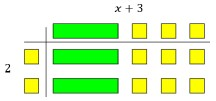• Instruction moves from concrete models of examples with integer coefficients to rational coefficients.
• Instruction includes providing and discussing tasks that involve students analyzing errors which helps students with the ability to self-correct misconceptions within their own work.

### Common Misconceptions or Errors

• Students may incorrectly apply the distributive property by multiplying the monomial to only one of the terms in the parentheses. To address this misconception, emphasize that it is the distributive property of multiplication over addition to help support student understanding.
• Students may incorrectly apply the rules of integers as they distribute when working with the operations of negative numbers and applying the distributive property of multiplication over addition.
• Students may incorrectly change the degree of the variable in order to simplify terms.

### Strategies to Support Tiered Instruction

• Teacher models examples using the area model for multiplication, showing that the monomial should be multiplied by each term of the polynomial.
• For example, the area model can be used to determine the product between 45 and 5 is 225. Once students understand how distribution works, teachers reintroduce variables and solve problems the same way.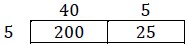• For example, the area model can be used to determine the product between $x$ −3 and 4 is 4$x$ −12.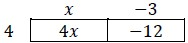• For example, the area model can be used to determine the product between 3$y$ and $y$ + 2$y$² is 3$y$² + 6$y$³.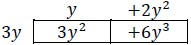• If teachers did not utilize algebra tiles in whole group instruction, algebra tiles could be used when solving problems using the distributive property. This will help students who incorrectly distribute the monomial to only one term of the polynomial.
• Teachers may color code each step of the problem so students can see the progression of distribution.
• For example, the expression 3($x$ + 7) is equivalent to 3$x$ +21.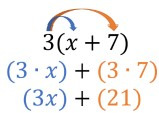• Instruction includes emphasizing that it is the distributive property of multiplication over addition to help support student understanding.

Students were working on the math problem −$\frac{\text{1}}{\text{2}}$$x$(4$x$ + 8). Kevin’s result is shown in the table below.
• Part A. Is Kevin’s answer correct?
• Part B. If no, explain his misconception and how he can correct his mistake. If yes, explain why he is correct and the steps he could have used.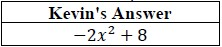A rectangle is given below. Note: The figure is not drawn to scale.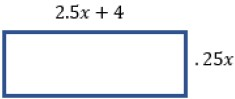• Part A. Find the area in terms of $x$.
• Part B. If the value of $x$ is 4 cm, what is the area of the rectangle in square centimeters?

### Instructional Items

Instructional Item 1
An expression is given below.
$\frac{\text{7}}{\text{8}}$$x$ ($\frac{\text{3}}{\text{4}}$$x$$\frac{\text{5}}{\text{6}}$)
What is the product of the expression?

Instructional Item 2
Find the product of 0.25$x$(0.55$x$ − 0.3).

*The strategies, tasks and items included in the B1G-M are examples and should not be considered comprehensive.

## Related Courses

This benchmark is part of these courses.
1200400: Foundational Skills in Mathematics 9-12 (Specifically in versions: 2014 - 2015, 2015 - 2022, 2022 and beyond (current))
1205050: M/J Accelerated Mathematics Grade 7 (Specifically in versions: 2014 - 2015, 2015 - 2020, 2020 - 2022, 2022 and beyond (current))
1205070: M/J Grade 8 Pre-Algebra (Specifically in versions: 2014 - 2015, 2015 - 2022, 2022 and beyond (current))
1204000: M/J Foundational Skills in Mathematics 6-8 (Specifically in versions: 2014 - 2015, 2015 - 2022, 2022 and beyond (current))
7812030: Access M/J Grade 8 Pre-Algebra (Specifically in versions: 2014 - 2015, 2015 - 2018, 2018 - 2019, 2019 - 2022, 2022 and beyond (current))

## Related Access Points

Alternate version of this benchmark for students with significant cognitive disabilities.
MA.8.AR.1.AP.2: Use the distributive property to multiply a monomial by a linear expression.

## Related Resources

Vetted resources educators can use to teach the concepts and skills in this benchmark.

## Student Resources

Vetted resources students can use to learn the concepts and skills in this benchmark.

## Parent Resources

Vetted resources caregivers can use to help students learn the concepts and skills in this benchmark.